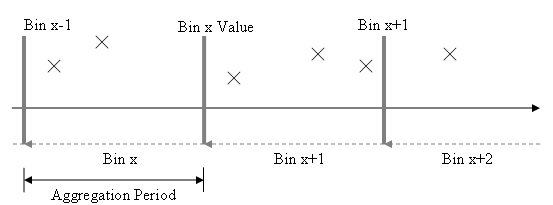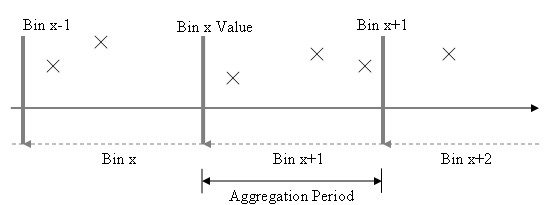Data Aggregation

Overview of Aggregation Period and Aggregation Function

The section of time is from the current pre-processed Data Point time (inclusive) to the last pre-processed Data Point time (exclusive). These sections of time are referred to as Bins. Any data lying within a Bin is considered in the bin’s aggregate value calculation.

In the configuration of the MCE, you can set a True/False flag to determine the core aggregation direction of the calculation engine. False implies that values in the bucket xx:xx:xx+1 tick to yy:yy:yy will be aggregated to time yy:yy:yy.

True (ie Forward aggregation) implies that values in the bucket xx:xx:xx to yy:yy:yy – 1 tick will be aggregate to time xx:xx:xx.

The following diagram illustrates the aggregation Bin concept when the flag is set to FalseAggregation Period defines the time between pre-processed Data Points, and therefore the size of each Bin.

When forward aggregation is set to True, then the bin is forward of the data point in the center as shown here:It is important to note that the bin is inclusive of the data point time, and exclusive of the bounding data point time

Aggregation Period

The Aggregation Period defines the periodicity of the data that will be stored in the Calculated Data table. By default the default Aggregation Period is set to None which means that Aggregation will not be used and a default Aggregation Period of 1 day will be used. I.e. a calculated value will be derived every day.

For Aggregation of half hourly electricity data to a Daily basis then the Aggregation Every value will be set to Daily. If you wish to Aggregate the data every hour then the Aggregation Every value will be set to hourly. Available Aggregation Periods of 1 second to 1 month are available.

In practice it is advised that the Aggregation Period is not set to a value less than the natural periodicity of the raw data. I.e. if the raw data has a periodicity of 15 minutes then the minimum Aggregation Period that should be set is also 15minutes.

If aperiodic raw data is used (i.e. there is no natural periodicity) then MCE will impose the periodicity defined by the Aggregation Period.

Aggregation Function

When the Aggregation eriod is anything other than None MCE uses this function to apply to the data that falls within the Aggregation Bucket. Eg Sum, Avg, First and Last.

If you wish to aggregate a half hour electricity meter from 48 half hour values to 1 daily sum then set the Aggregation Period to Daily and the Aggregation Function to Sum